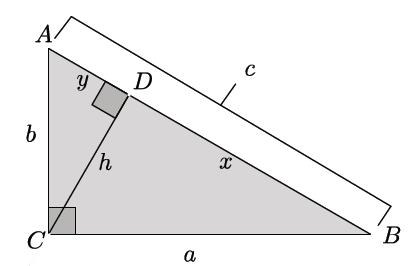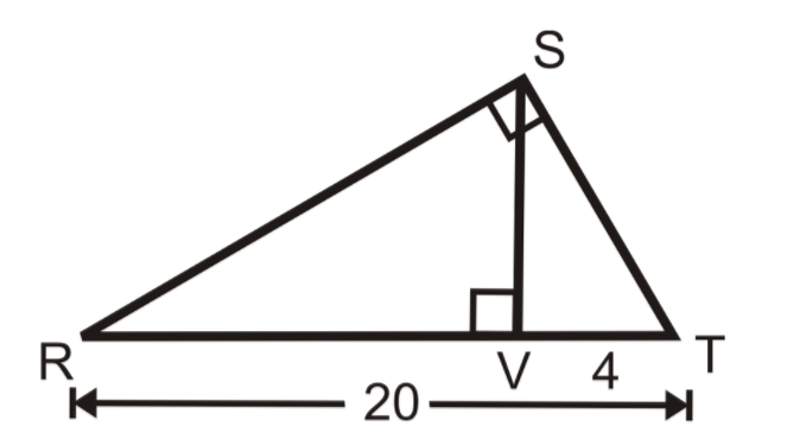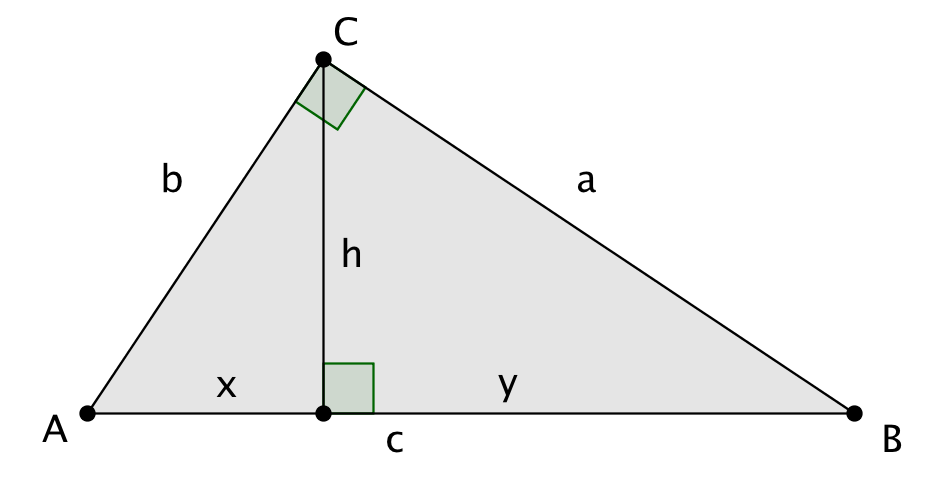# Right Triangles and Trigonometry

## Objective

Define and prove the Pythagorean theorem. Use the Pythagorean theorem and its converse in the solution of problems.

## Common Core Standards

### Core Standards

?

• G.SRT.B.4 — Prove theorems about triangles. Theorems include: a line parallel to one side of a triangle divides the other two proportionally, and conversely; the Pythagorean Theorem proved using triangle similarity.

?

• 8.EE.A.2

• 8.G.B.6

• 8.G.B.7

• 8.G.B.8

## Criteria for Success

?

1. Define the Pythagorean theorem as, “If a triangle is a right triangle, then the sum of the squares of the two shorter sides is equal to the square of the hypotenuse.”
2. Define the converse of the Pythagorean theorem as, “If the sum of the squares of two shorter sides is equal to the square of the third side, then the triangle is a right triangle.”
3. Prove the Pythagorean theorem using the similarity of the two triangles formed by subdividing the larger triangle using an altitude.
4. Use the Pythagorean theorem to find missing measures in right triangles.
5. Use altitudes and the Pythagorean theorem to identify missing measures (directly or algebraically) and in the development of proofs.

## Tips for Teachers

?

• This lesson formalizes students’ understanding of the Pythagorean theorem from Grade 8 Geometry.
• Anchor Problem #1 will require the teacher to do a significant amount of modeling to prove the Pythagorean theorem and its converse. Reference EngageNY, Geometry, Module 2, Lesson 24: Teacher Version for more guidance on this.
• Students may need to review simplifying radicals before they can fully access this lesson. It is recommended to spend time outside of class building this specific skill.

## Anchor Problems

?

### Problem 1

Given the subdivided right triangle below, show that ${a^2+b^2=c^2}$1. Write an expression in terms of $c$ for $x$ and $y$.
2. Write a similarity statement for the three right triangles in the diagram.
3. Write a ratio that shows the relationship between side lengths of two of the triangles.
4. Prove the Pythagorean theorem.
5. Prove the converse of the Pythagorean theorem.

#### References

EngageNY Mathematics Geometry > Module 2 > Topic D > Lesson 24Image from Teacher Version: Opening

Geometry > Module 2 > Topic D > Lesson 24 of the New York State Common Core Mathematics Curriculum from EngageNY and Great Minds. © 2015 Great Minds. Licensed by EngageNY of the New York State Education Department under the CC BY-NC-SA 3.0 US license. Accessed Dec. 2, 2016, 5:15 p.m..

### Problem 2

Find ${RS}$.#### References

Image from Example 3

8.3 Using Similar Right Triangles is made available by CK-12 under the CC BY-NC 3.0 license. © CK-12 Foundation 2017. Visit them at ck12.org. Accessed Feb. 28, 2018, 11:10 a.m..

## Problem Set

?

The following resources include problems and activities aligned to the objective of the lesson that can be used to create your own problem set.

?

### Problem 1

In each row in the table below are the lengths of the legs and hypotenuse of different right triangles. Find the missing side lengths in each row in simplest radical form.

 ${\mathrm{Leg}_1}$ ${\mathrm{Leg}_2}$ Hypotenuse 15 25 15 36 3 7 100 200

#### References

EngageNY Mathematics Geometry > Module 2 > Topic D > Lesson 24Problem Set, Question #1

Geometry > Module 2 > Topic D > Lesson 24 of the New York State Common Core Mathematics Curriculum from EngageNY and Great Minds. © 2015 Great Minds. Licensed by EngageNY of the New York State Education Department under the CC BY-NC-SA 3.0 US license. Accessed Dec. 2, 2016, 5:15 p.m..

### Problem 2

In right triangle ${ABC}$ with ${\angle C}$ a right angle, an altitude of length $h$ is dropped to side ${\overline{AB}}$ into segments of length $x$ and $y$. Use the Pythagorean theorem to show $h^2=xy$#### References

EngageNY Mathematics Geometry > Module 2 > Topic D > Lesson 24Problem Set, Question #5

Geometry > Module 2 > Topic D > Lesson 24 of the New York State Common Core Mathematics Curriculum from EngageNY and Great Minds. © 2015 Great Minds. Licensed by EngageNY of the New York State Education Department under the CC BY-NC-SA 3.0 US license. Accessed Dec. 2, 2016, 5:15 p.m..